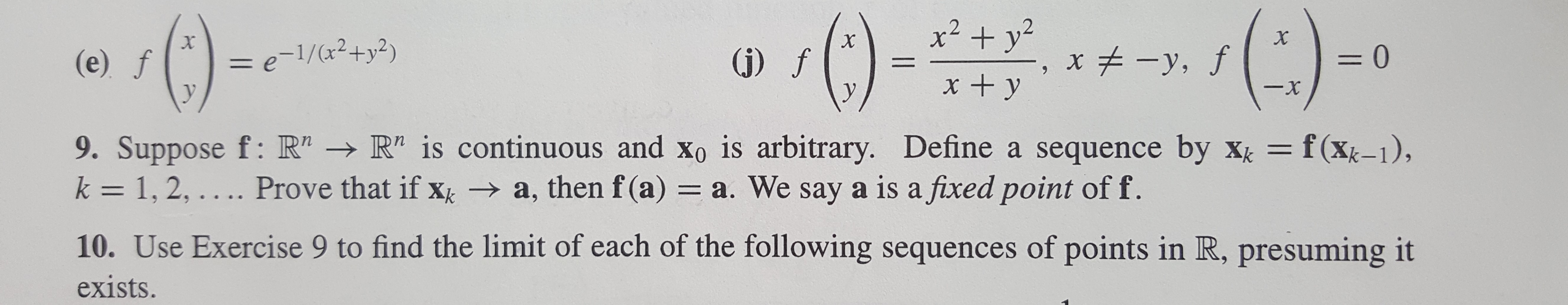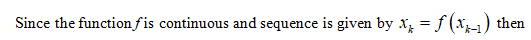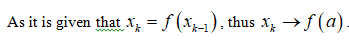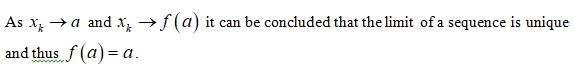# x2 +yx, fX= e-1/(x2+y2)= 0(j) f(e) f1x + y-xy9. Suppose f: R" R" is continuous and Xo is arbitrary. Define a sequence by xk f(Xk-1),k 1, 2,.... Prove that if x a, then f (a) = a. We say a is a fixed point of f.10. Use Exercise 9 to find the limit of each of the following sequences of points in R, presuming itexists.

Question
39 views

I need help for problem 9. Thanks!help_outlineImage Transcriptionclosex2 +y x , f X = e-1/(x2+y2) = 0 (j) f (e) f 1 x + y -x y 9. Suppose f: R" R" is continuous and Xo is arbitrary. Define a sequence by xk f(Xk-1), k 1, 2,.... Prove that if x a, then f (a) = a. We say a is a fixed point of f. 10. Use Exercise 9 to find the limit of each of the following sequences of points in R, presuming it exists. fullscreen
check_circle

star
star
star
star
star
1 Rating
Step 1Step 2Step 3...

### Want to see the full answer?

See Solution

#### Want to see this answer and more?

Solutions are written by subject experts who are available 24/7. Questions are typically answered within 1 hour.*

See Solution
*Response times may vary by subject and question.
Tagged in

### Math